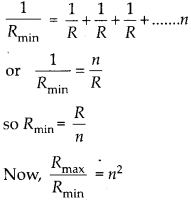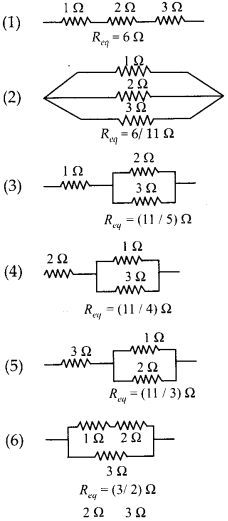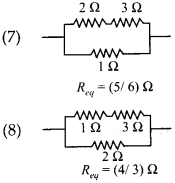Enlightened

# Question 20: NCERT Solutions for 12th Class Physics: Chapter 3-Current Electricity

• 0

Question 20: NCERT Solutions for 12th Class Physics: Chapter 3-Current Electricity

(a) Given n resistors each of resistance R, how will you combine them to get the

• maximum
• minimum effective resistance? What is the ratio of the maximum to minimum resistance?

(b) Given the resistances of 1 Ω, 2Ω, 3Ω, how will be combine them to get an equivalent resistance of

• (11/3) Ω
• (11/5) Ω,
• 6 Ω,
• (6/11) Ω?

(c) Determine the equivalent resistance of network shown in figure.Share

1. Solution:

(a)

• For maximum effective resistance, all the resistors should be joined in series. Rmmax = R + R + R+……..n or Rmax = nR
• For minimum effective resistance, all the resistors should be joined in parallel.(b) All possible combinations with resistances 1 Ω, 2 Ω and 3 Ω areSo, the combinations for the desired results can be selected.Check the complete chapter with solutions.

NCERT Solutions for 12th Class Physics: Chapter 3-Current Electricity

• 0